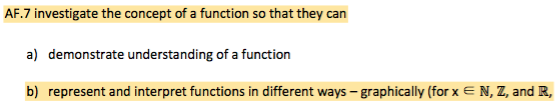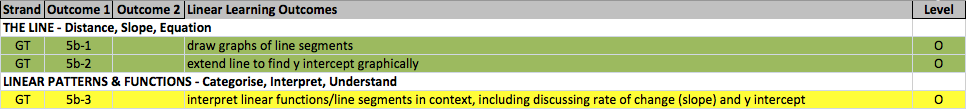# PD6-Post 01

Decomposition of Learning Outcomes into Topics and Concepts

The new Junior Cycle Mathematics syllabus consists of four stands described as learning outcomes (LOs) (NCCA, 2017). This research project chose eight specific LOs from Co-ordinate Geometry of the Line, Patterns, and Functions for their interconnectedness. The first step in the design and development of the domain model artefacts involved the use of Microsoft Excel. This application was used to decompose the eight syllabus LOs into fine-grained LOs and concepts, and later into topics. The rationale for unpacking the LOs is that fine-grained domain models are required for precise adaptation (Sosnovsky & Brusilovsky, 2015).

(Ahmad et al., 2004) state that the domain model deals with “…the decomposition of concepts in a structured hierarchy of sub-concepts and atomic information…” In this research project, the initial target objects for decomposition were LOs. This is because the syllabus specifies LOs rather than concepts or topics. The decomposition process executed in this research project can be described by three distinct phases.

Phase 1 – Unpacking the 8 Syllabus LOs to 45 Finely Grained LOs
An example of this unpacking process is illustrated by taking one of the syllabus LOs relating to Patterns, AF.1a (Figure 3) and unpacking it into the six LOs AF 1a-1 to AF 1a-6 (Figure 4).Figure 3: One Syllabus Learning Outcome: Patterns (AF.1a)Figure 4: Six Unpacked Learning Outcomes (AF 1a-1 to AF 1a-6)

Two further examples of unpacking a syllabus LO relate to Functions (Figure 5 and Figure 6) and Co-ordinate Geometry of the Line (Figure 7 and Figure 8).Figure 5: One Syllabus Learning Outcome: Functions (AF.7b)Figure 6: Six Unpacked Learning Outcomes (AF 7b-1 to AF 7b-8)Figure 7: One Syllabus Learning Outcome: The Line (GT.5b)Figure 8: Three Unpacked Learning Outcomes (GT 5b-1 to GT 5b-3)

All 45 unpacked LOs can be seen in Figure 11. A colour scheme was used whereby similar representations of concepts have similar colours. For example, orange is used for ‘Tables’ and green is used for ‘Graphs’. The reason for doing this was that it helped to build the hierarchies of concepts used in the Mindomo artefact and to make connections between topics and concepts in the Rhumbl Maps artefact.

Phase 2 – Unifying the Learning Outcomes for Patterns and Functions
An example of the unification process can be seen in Figure 9. The six LOs relating to Patterns (Figure 4) are paired up with six corresponding LOs relating to Functions (Figure 6). For example, there is now a single unified LO from AF 1a-1 described as “represent linear patterns and functions in tables” (Figure 9).Figure 9: Six Pairs of Unified Learning Outcomes (AF 1a-1 to AF 7b-8)

Phase 3 – Distilling the Learning Outcomes for The Line, Linear Patterns, Linear Functions
The distilling process involved removing any LOs that did not have the words ‘linear’ or ‘line’ from the 45 unpacked LOs created in Phase 1 (Figure 11).

Some of the ‘Linear’ LOs arrived at in Phase 3 can be seen by combining the three LOs in The Line and Linear Patterns & Functions (Figure 8) with the four LOs in Linear Patterns & Functions (Figure 9). This gives the seven ‘Linear’ LOs that can be seen in Figure 10.Figure 10: Seven Linear Learning Outcomes (GT 5b-1 to GT 5b-3)